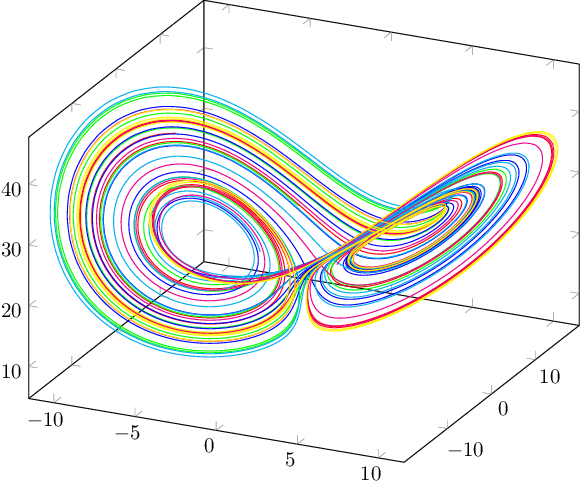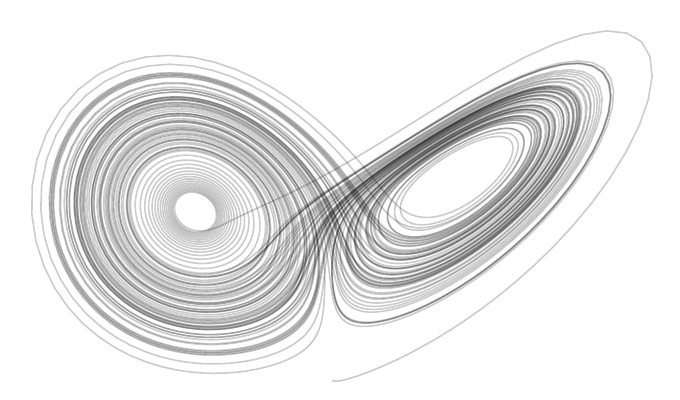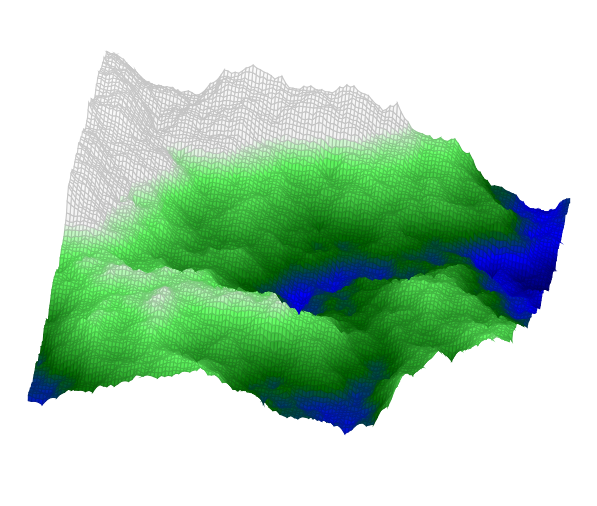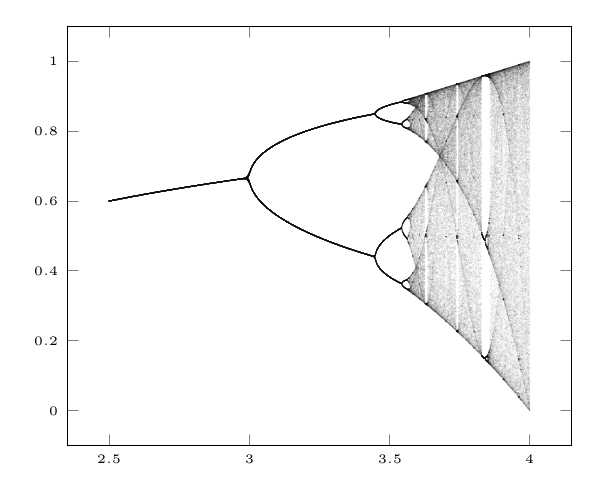﻿ Dynamic systems, bifurcations, procedural worlds | TeXblog

## Dynamic systems, bifurcations, procedural worlds

I’m using pgfplots a lot, so I will share some examples today.

Here, I benefit from these features of pgfplots, going beyond base TikZ:

• Simple plotting with 3d coordinates and axonometric projection
• Presentation of required coordinate axes
• Reading in files with externally calculated data

In any case we can use Lua for calculating data. Lua generates the TeX commands for printing, which will be processed in a pgfplots axis environment.

Here are the samples, just click on it to get to the corresponding thread on TeXwelt with full source code.

## Lorenz attractor (dynamic system)

While I posted a Python calculated version on TeXwelt.de, Henri added one, which bases on LuaTeX. Let’s see his picture at first:Of pgfplots I used the transparency feature besides the standard 3d plot, so I got an impression of the density:Once we calculated the data, the code is simple:

\documentclass[border=10pt]{standalone} \usepackage{pgfplots} \begin{document} \begin{tikzpicture} \begin{axis}[ xmin = -25, xmax = 25, ymin = -25, ymax = 25, zmin = 0, zmax = 50, hide axis, ] \addplot3[mark=none, mesh, shader=interp, color=black, opacity=0.2] file { lorenz.dat }; \end{axis} \end{tikzpicture} \end{document}

## Fractal landscapes – the base for producing procedural worlds

Between adjacent points, new points will be calculated, with random but limited variation. Finally we will get a mountainous landscaoe. The calculated points get color according to their height: blue for sea level and below, green for mountains and white above the snowline.Next step: specify nice starting values, for beginning with a certain base structure, such as an island in the water.

## Feigenbaum diagram (bifurkations)

This is a classic of the chaos theory und closely related to the Mandelbrot set. Also here, we use transparency for an impression of the point density.I often started such topics on TeXwelt.de. LaTeX support for thesis writers is not the only talking point there. It became established, that TeX connoisseurs post their ideas in shape of a question, often themselves posting the first answer, opening a discussion. The final goal is a knowledge database, built on top of questions and answers.

08. July 2014 by stefan